### Chemical and Process Engineering Resources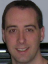## Measure Discharge Flow from a Horizontal Pipe

Nov 08 2010 01:30 PM | Chris Haslego in Calculations and TipsEver been confronted with the exit of a horizontal pipe full of flow?Â  Wouldn't it be nice if there was a simple method to determine the flow rate in the pipe?Â  Here's a method that may help.

This works surprisingly well and is very easy to do. All measurements are in inches. The formula is invalid for any other units. Also, the discharging pipe must be full of liquid.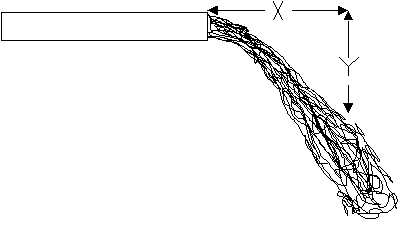Figure 1: Schematic Diagram

Using a yardstick, measure any convenient distance X across the top of the liquid discharge (Figure 1).Â Â  Then measure the corresponding drop distance Y from the end of X to the top of the liquid. Measure the diameter of the pipe in inches.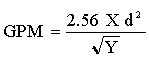Eq. (1)

For example â€“ measure a distance, X, of 24 inches across the top of the flowing discharge from the end of a 2 inch diameter pipe. Then measure vertically to the top of the liquid discharge, Y, say it is 25 inches. Then by the formula, the flow in the pipe in gallons per minute is:

2.56 times 24 times 2 squared divided by the square root of 25 =

2.56 times 96 divided by 5 =

49.15 say 50 GPM

References

1. "Engineering Handbook of Conversion Factors" by Combustion Engineering, 1977, pg.142.

### Calculations and Tips Articlesaprsunya
What is the basis of this calculation? Can you elaborate?Chris Haslego
This tip was provided by a visitor quite some time ago. I recall that he had validated it as fairly accurate within the constraints provided. I don't have the reference that he provided, but the basis for the equation may be found there.Guest
The factor should be 2.83, not 2.56:

x= velocity (in/s) * t
y= 1/2 * 386.088 in/s^2 * t^2 (gravitational acceleration)
x/sqrt(y) = velocity (in/s) /13.894 (time cancels out)
velocity = flow (gpm) * 231 in^3/gal * 1 min/60 sec/(.25*d^2*pi) (change units and divide by area)
velocity = flow /d^2 *4.9 (simplify)
x/sqrt(y) = 0.353 * flow/d^2 (replace velocity from prior equation and simplify)
flow (GPM) = X * d^2 * 2.83 / sqrt(Y) (solve for flow)dhrubabasu2013

Whats the basis of taking Y...suppose the distance from ground to pipe that the liquid is falling is very high..

### Forum Quick Links

•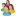Industrial Forum

•Student Forum

•Refining Forum

•Simulation Forum

•Relief Devices Forum

•Tank Venting Forum

• ### Article Navigation

•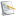Separation Technology

•Calculations and Tips

•Fluid Flow

•Heat Transfer

•Maintenance and Repair

•Utilities

•Safety and Pressure Relief

•Bulk Solids

•Process and Reactions

•Energy

•Other Topics

•For Students

•Physical Properties

•Archived Articles